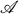Hostname: page-component-7d684dbfc8-zgpz2 Total loading time: 0 Render date: 2023-09-29T09:51:16.727Z Has data issue: false Feature Flags: { "corePageComponentGetUserInfoFromSharedSession": true, "coreDisableEcommerce": false, "coreDisableSocialShare": false, "coreDisableEcommerceForArticlePurchase": false, "coreDisableEcommerceForBookPurchase": false, "coreDisableEcommerceForElementPurchase": false, "coreUseNewShare": true, "useRatesEcommerce": true } hasContentIssue false

# Almost Convergence, Summability And Ergodicity

Published online by Cambridge University Press:  20 November 2018

## Extract

Core share and HTML view are not possible as this article does not have html content. However, as you have access to this content, a full PDF is available via the ‘Save PDF’ action button.

The notion of almost convergence introduced by Lorentz  has been generalized in several directions (see, for example [1; 8; 11 ; 14; 17]). I t is the purpose of this paper to give a generalization based on the original definition in terms of invariant means. This is effected by replacing the shift transformation by an "ergodic" semigroupof positive regular matrices in the definition of invariant mean. The resulting "- invariant means" give rise to a summability method which we dub-almost convergence.

Type
Research Article
Information
Canadian Journal of Mathematics , April 1974 , pp. 372 - 387

## References

1. Atalla, R., On the inclusion of a bounded convergence field in the space of almost convergent sequences, Glasgow Math. J. 31 (1972), 8290.CrossRefGoogle Scholar
2. Borwein, D., A logarithmic method of summability, J. London Math. Soc. 38 (1958), 212220.CrossRefGoogle Scholar
3. Chou, C., The multipliers for the space of almost convergent sequences, Illinois J. Math. 16 (1972), 687694.CrossRefGoogle Scholar
4. Chou, C. and Duran, J. P., Multipliers for the space of almost-convergent functions on a semigroup, Proc. Amer. Math. Soc. 39 (1973), 125128.CrossRefGoogle Scholar
5. Day, M. M., Means for the bounded functions and ergodicity of bounded representations of semigroups, Trans. Amer. Math. Soc. 69 (1950), 276291.CrossRefGoogle Scholar
6. Dean, D. and Raimi, R. A., Permutations with comparable sets of invariant means, Duke Math. J. 27 (1960), 467480.CrossRefGoogle Scholar
7. Eberlein, W. F., Abstract ergodic theorems and weak almost periodic functions, Trans. Amer. Math. Soc. 67 (1949), 217240.CrossRefGoogle Scholar
8. Eberlein, W. F., Banach-Hausdorff limits, Proc. Amer. Math. Soc. 1 (1950), 662665.CrossRefGoogle Scholar
9. Eberlein, W. F., On Hölder summability of infinite order, Notices Amer. Math. Soc. 19 (1972), A-164; Abstract #691-46-21.Google Scholar
10. Fuchs, W. H. J., On the “collective Hausdorff method”, Proc. Amer. Math. Soc. 1 (1950), 2630.Google Scholar
11. Garten, V. and Knopp, K., Ungleichungen zwischen Mittlewerten von Zahlenfolgen und Funktionen, Math. Z. 42 (1937), 365388.CrossRefGoogle Scholar
12. Hardy, G. H., Divergent series (Oxford University Press, Oxford, 1949).Google Scholar
13. Jerison, M., On the set of generalized limits of bounded sequences, Can. J. Math. 9 (1957), 7989.CrossRefGoogle Scholar
14. King, J. P., Almost summable sequences, Proc. Amer. Math. Soc. 17 (1966), 12191225.CrossRefGoogle Scholar
15. Lorentz, G. G., A contribution to the theory of divergent sequences, Acta Math. 80 (1948), 167190.CrossRefGoogle Scholar
16. Petersen, G. M., Almost convergence and uniformly distributed sequences, Quart. J. Math. Oxford Ser. 2, 7 (1956), 188191.Google Scholar
17. Raimi, R. A., On Banach's generalized limits, Duke Math. J. 26 (1959), 1728.CrossRefGoogle Scholar
18. Raimi, R. A., Invariant means and invariant matrix methods of summability, Duke Math. J. 33 (1966), 112.CrossRefGoogle Scholar
19. Schaefer, P., Matrix transformations of almost convergent sequences, Math. Z. 112 (1969), 321325.CrossRefGoogle Scholar